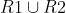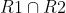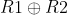# DM - Functions & Relations -Q11

+1 vote

Suppose that R1 and R2 are two equivalence relations on non empty set A then which of the following statements is necessarily true?.is an equivalence relation.is an equivalence relation.is an equivalence relation

Which of the following statements is true?

(A). Only(B). Only(C). Onlyand(D). Onlyasked Jun 24
reshown 6 days agois not reflexive.
Please note that  is symmetric difference operator.
For example Domain = {1,2,3}
R1 = {(1,1),(2,2),(3,3) }
R2= {(1,1),(2,2),(3,3),(1,2),(2,1)}

is {(1,2),(2,1) }, which is not reflexive.answered 4 days ago by (112,390 points)